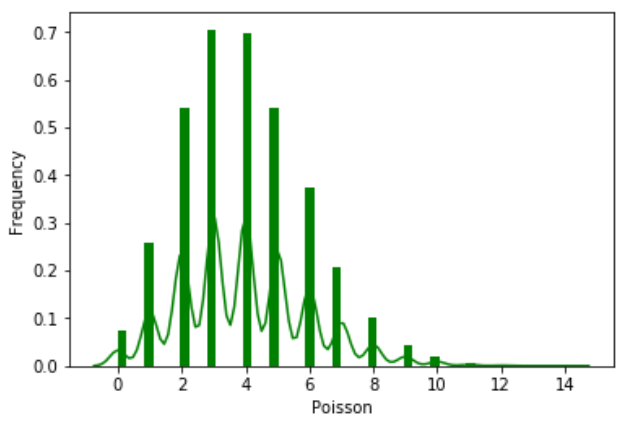# Python – Poisson Distribution

A Poisson distribution is a distribution which shows the likely number of times that an event will occur within a pre-determined period of time. It is used for independent events which occur at a constant rate within a given interval of time. The Poisson distribution is a discrete function, meaning that the event can only be measured as occurring or not as occurring, meaning the variable can only be measured in whole numbers.

We use the seaborn python library which has in-built functions to create such probability distribution graphs. Also the scipy package helps is creating the binomial distribution.

```from scipy.stats import poisson
import seaborn as sb

data_binom = poisson.rvs(mu=4, size=10000)
ax = sb.distplot(data_binom,
kde=True,
color='green',
hist_kws={"linewidth": 25,'alpha':1})
ax.set(xlabel='Poisson', ylabel='Frequency')```

Its output is as follows −